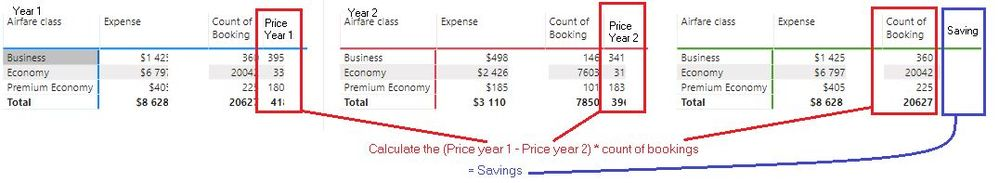cancel
Showing results for
Did you mean:Frequent Visitor

## Subtracting between measurements

Hi,

I'm struggling with this case for quite some time now, hopefully this community got some tricks up their sleves...

So what i wanna do is calculate the savings by subtracting the price year 2 from the price year 1 and multiplying it with the current years bookings.

Another thing that might be good to know is that the prices from year 1 and 2 are measures in the same table.Thanks for any support!

3 REPLIES 3Anonymous
Not applicable

final =

var sub=[price1]-[price2]

Return

[Count of booking]*Sub

Thanks,

Pravin Wattamwar

If it resolves your problem mark it as solution and give kudos.Frequent Visitor

Thanks for the quick answer Pravin!

What i was not to clear about was that the measures price1 and price2 are the same. Is it perhaps possible to add a filter to your code?

ThanksCommunity Champion

Hi @dannyd

try to

``````Measure =
var _year1 = 2018
var _year2 = 2019
RETURN
(CALCULATE([price]; YEAR('Table'[Date])=_year1) - CALCULATE([price]; YEAR('Table'[Date])=_year2)) * CALCULATE(Count('Table'[Booking]))``````

do not hesitate to give a kudo to useful posts and mark solutions as solution

do not hesitate to give a kudo to useful posts and mark solutions as solution